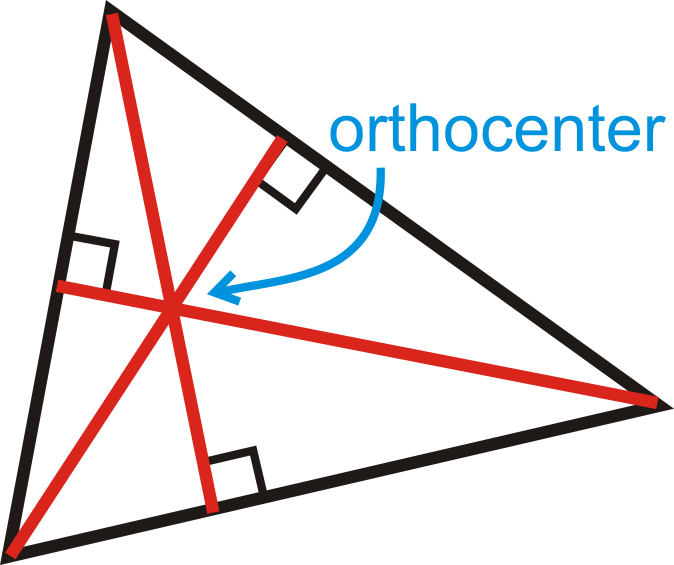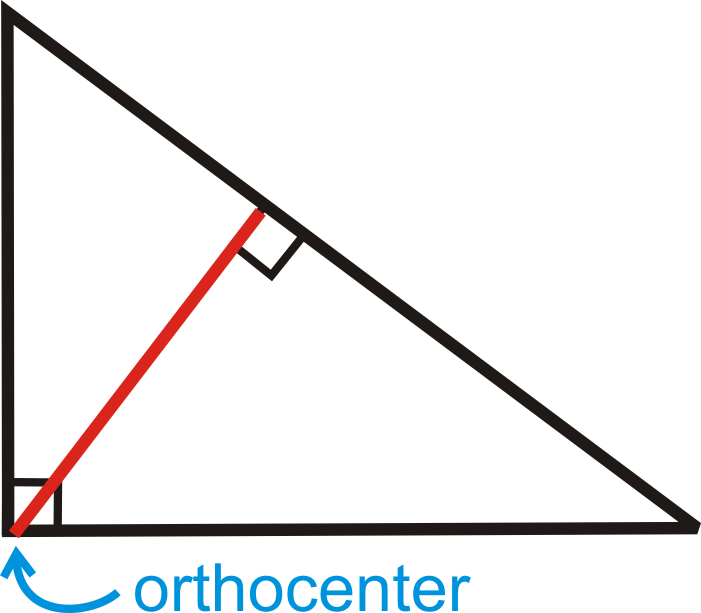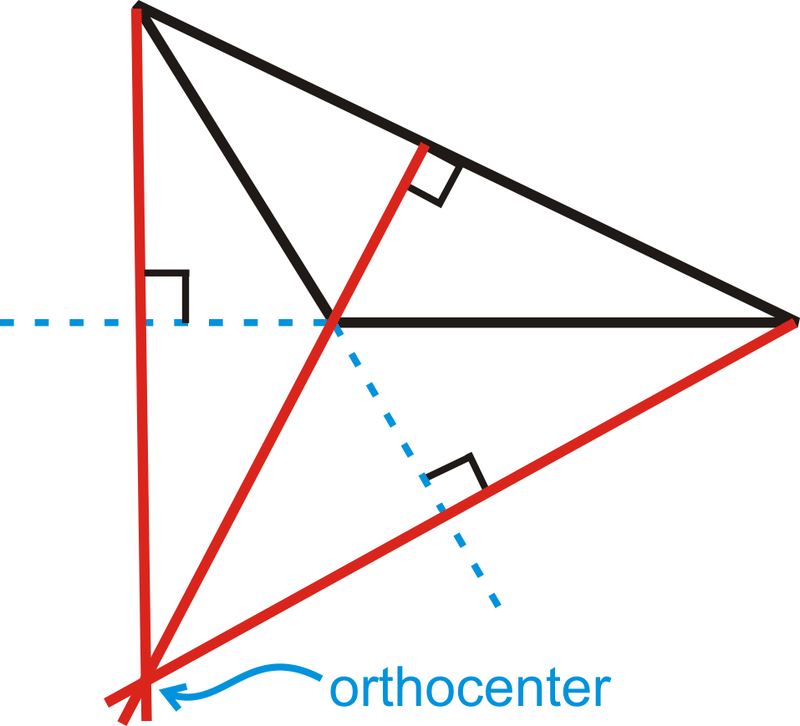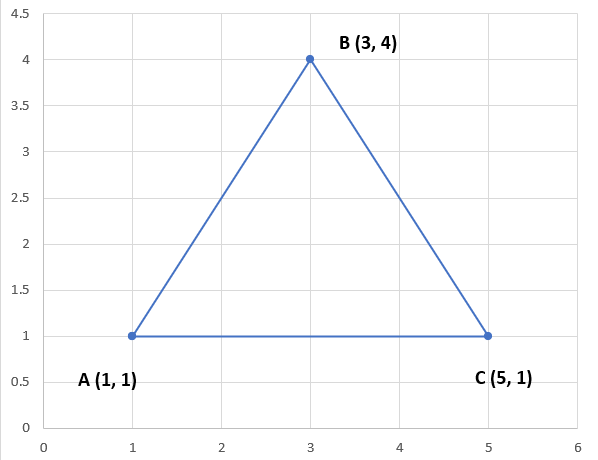# Orthocentre

The 3 altitudes of a triangle intersect at a common point called the orthocentre. Its location depends on the type of triangle; unlike the median, it can be located either inside, outside, or on the vertex of a triangle.

### Acute TriangleThe orthocentre is located on the inside of the triangle.

### Right TriangleThe orthocentre is located on the vertex of the right angle.

### Obtuse TriangleThe orthocentre is located on the outside of the triangle.

## Steps to Calculate Orthocentre

1. Calculate the slope of at least 2 side lengths of the triangle using the formula $$m = \cfrac{y2 - y1}{x2 - x1}$$
2. Using the slopes of the side lengths, find the slopes of the altitudes by calculating the perpendicular slopes using the formula $$m = \cfrac{-1}{m}$$
3. Use the slope-point formula $$y - y₁ = m(x - x₁)$$ to determine the altitudes. $$m$$ represents the slope of the altitude and $$(x₁, y₁)$$ represents the point it connects to
4. Set the altitudes equal to each other to solve for the x-coordinate of the orthocentre
5. Plug the x-value into one of the altitude formulas to solve for the y-coordinate

Example

Find the orthocentre of the following triangle:We can find the slopes of 2 side lengths of the triangle. In this instance, we will find the slopes of sides $$\text{AB}$$ and $$\text{BC}$$ with $$\text{A}$$ acting as Point 1, $$\text{B}$$ acting as Point 2 and $$\text{C}$$ acting as Point 3:

m(ᴀʙ) $$= \cfrac{y₂ - y₁}{x₂ - x₁}$$
m(ᴀʙ) $$= \cfrac{4 - 1}{3 - 1}$$
m(ᴀʙ) $$= \cfrac{3}{2}$$

m(ʙᴄ) $$= \cfrac{y₃ - y₂}{x₃ - x₂}$$
m(ʙᴄ) $$= \cfrac{1 - 4}{5 - 3}$$
m(ʙᴄ) $$= \cfrac{-3}{2}$$

Now that we have the slopes, we can determine the slopes of the altitudes by calculating the inverse slopes:

m(altitude)AB $$= \cfrac{-1}{3/2}$$
m(altitude)AB $$= \cfrac{-2}{3}$$

m(altitude)BC $$= \cfrac{-1}{-3/2}$$
m(altitude)BC $$= \cfrac{2}{3}$$

Since we have the slopes of the altitudes, we can plug these values into the slope-point formula to determine the altitudes For side length $$\text{AB}$$, we will be using Point $$\text{C}$$. For side length $$\text{BC}$$, we will be using Point $$\text{A}$$:

altitudeAB: $$y - 1 = \cfrac{-2}{3}(x - 5)$$
altitudeAB: $$y - 1 = \cfrac{-2}{3}x + \cfrac{10}{3}$$
altitudeAB: $$y = \cfrac{-2}{3}x - \cfrac{10}{3} + \cfrac{3}{3}$$
altitudeAB: $$y = \cfrac{-2}{3}x + \cfrac{13}{3}$$

altitudeBC: $$y - 1 = \cfrac{2}{3}(x - 1)$$
altitudeBC: $$y - 1 = \cfrac{2}{3}x - \cfrac{2}{3}$$
altitudeBC: $$y = \cfrac{2}{3}x - \cfrac{2}{3} + \cfrac{3}{3}$$
altitudeBC: $$y = \cfrac{2}{3}x + \cfrac{1}{3}$$

Now that we have both altitudes, we can determine the location of the orthocentre. We can set the altitudes equal to each other to solve for $$x$$.

$$\cfrac{-2}{3}x + \cfrac{13}{3} = \cfrac{2}{3}x + \cfrac{1}{3}$$
$$\cfrac{-2}{3}x - \cfrac{2}{3}x = \cfrac{1}{3} - \cfrac{13}{3}$$
$$(\cfrac{-3}{4})(\cfrac{-4}{3}x) = (\cfrac{-3}{4})(\cfrac{-12}{3})$$
$$x = \cfrac{36}{12} = 3$$

Now we can plug the x-coordinate into one of the altitude formulas to solve for $$y$$:

$$y = (\cfrac{-2}{3})(3) + \cfrac{13}{3}$$

$$y = \cfrac{-6}{3} + \cfrac{13}{3}$$

$$y = \cfrac{7}{3}$$

Therefore, we can determine that the location of the orthocentre is $$(3, \(\cfrac{7}{3}$$)\).

Determine the orthocentre of △$$\text{CRT}$$ with coordinates $$\text{C}(1,8)$$, $$\text{R}(3, 12)$$ and $$\text{T}(6,2)$$.